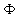NATIONAL ACADEMY OF SCIENCES OF UKRAINE PIDSTRYHACH INSTITUTE OF APPLIED PROBLEMS OF MECHANICS AND MATHEMATICS

Scientific Journal
"MATHEMATICAL METHODS and PHYICOMECHANICAL FILDS"

Year 2002, Vol.45
 Vol. 45, No.3, L'viv 2002 CONTENTS Dzhaluk N. S., Petrychkovych V. M. On common monic divisors of polynomial matrices with given canonical diagonal form 7 Prokip V. M. About structure of some sets of divisors of matrices over the principal ideal domain 14 Szavarovs’kyj B. Z On classification to within similarity of class of matrix pairs of the sixth order 22 Shchedryk V. P. Non-associated matrices with standard-skeleton 32 Podlevs’kyj B. M. One method of determination of amount of multiple roots of algebraic polynomials 45 Gavryliv O. S., Ostapovych B. S.,             Chabanyuk Ya. M. On the question of contractive semi-group on Cartesian products of abstract Wiener spaces 51 Sushch V. N. On some differential-difference analogue of mixed problem for wave equation 54 Tymoshchuk P. V., Stoliarchuk R. R. Mathematical macromodeling of nonlinear systems by integral-differential and corresponding discrete equations 62 Bomba A. Ya., Kashtan S. S. Nonlinear inversions of boundary-value problems on conformal mappings with controlling potential 69 Burak Ya. Yo., Chernukha O. Yu., Moros H. I. On correctness conditions for one class of ­boundary-value problems for admixture mass transfer by two ways 77 Savula Ya. G., Dyiak ˛. ˛., Dudash Î. ˛. Heterogeneous numerical scheme of domain decomposition technique to study plasticity problems 85 Yatskiv O. I., Shvets’ R. M. Construction of solution for mechano-diffusion problem on two-component diffusive saturation and stressed state of layered cylinder 91 Vasylenko Ŕ. T., Sudavtsova G. K. Stressed state of inhomogeneous orthotropic shells of revolution under action of asymmetric centrifugal loads 103 Vahin P. P., Malets R. B., Shynkarenko G. Ŕ. Thermoelastic analysis of flexible shift shells in quasistatic approximation 108 Kit H. S., Stankevych V. Z. Releigh wave diffraction on surface crack in half-space 118 Zavrazhina T. V. Influence of elastic pliability of manipulator links on precision of its positioning. I. Mathematical models of dynamics 124 Matviychuk K. S. Sufficient technical stability conditions of forced dynamic states in control systems with variable structure 134 Domans’kyy P. P., Soroka O. I. Optimization of form of highly supported pivots in problems of their stability in two measures 148 Terlec’kyy R. F. Thermomechanical behavior model of multicomponent deformable low electroconductivity solids in electromagnetic field. II. Statistical formulation of terms for electromagnetic field factor action on multicomponent solids 155 Kondrat V. F. Averaged magnetic field and mechanical stresses at magneto­elastic vibrations of electroconductive layer 169